# 超宽带小型圆极化天线的设计Design of an UWB Compact Circular Polarized Antenna

DOI: 10.12677/JA.2019.81001, PDF, HTML, XML, 下载: 462  浏览: 1,226

Abstract: An UWB compact circular polarized antenna is presented in this paper. The H slot is used as aper-ture to couple energy to patch antenna. This antenna has some advantages as wideband, small volum and performance in circularly polarizing, so it has broad appliance prosperity. This antenna operates from 2.2 GHz to 2.4 GHz, the relative bandwidth is 28.9% and the maximum gain is 5.74 dB. This antenna can be used not only a single antenna but element antenna in the array antenna. It can be used in navigation and satellite communication.

1. 引言

2. 天线单元设计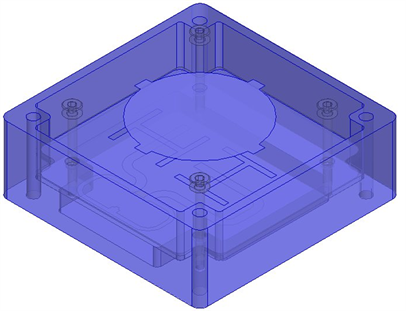Figure 1. The structure of antenna

$r=\frac{{r}_{有效}}{\sqrt{1+2H/\text{π}a{\epsilon }_{r}\left[\mathrm{ln}\left(\frac{\text{π}a}{2H}\right)+1.7726\right]}}$ (1)

${r}_{有效}=\frac{{{X}^{\prime }}_{np}c}{2\text{π}f\sqrt{{\epsilon }_{r}}}$ (2)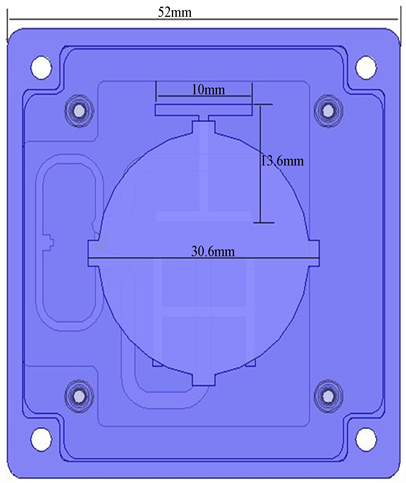Figure 2. The size of the structure

3. 功分网络设计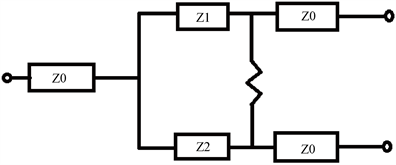Figure 3. The circuit model of the Wilkinson

4. 天线仿真与测试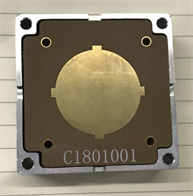Figure 4. The material of object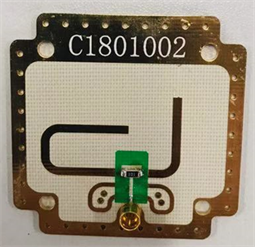Figure 5. The net of feed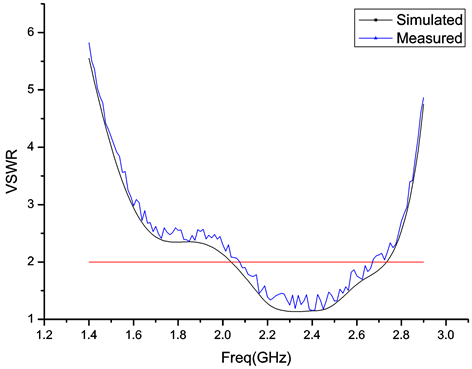Figure 6. The S11 of antenna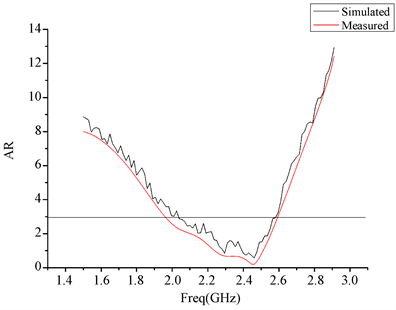Figure 7. The AR of antenna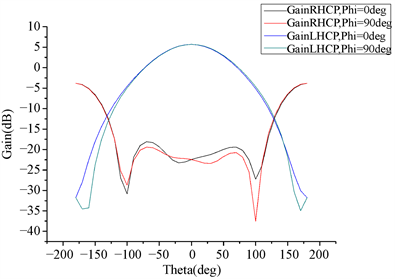Figure 8. The Radiation pattern of antennaFigure 9. The value of gain with the frequency

5. 结论

  Deschamps, G.A. (1953) Microstrip Microwave Antennas. USAF Symposium on Antennas.  鲍尔. 微带天线[M]. 梁联倬, 冠廷辉, 译. 北京: 电子工业出版社, 1985.  Rathi, V., Kumar, G. and Ray, K.P. (1996) Improved Coupling for Aperture Coupled Microstrip Antennas. IEEE Transactions on Antennas and Propagation, 44, 1196-1198. https://doi.org/10.1109/8.511831  王玉峰, 常雷, 何小煜. 圆极化天线技术[M]. 北京: 国防工业出版社, 2017.  叶云裳. 航天器天线[M]. 北京: 中国科学技术出版社, 2007.  钟顺时. 微带天线理论与应用[M]. 西安: 西安电子科技大学出版社, 1991.  Pozar, D.M. 微波工程[M]. 张肇仪, 等, 译. 北京: 电子工业出版社, 2006.  魏文元, 宫德明, 陈必森. 微波工程[M]. 北京: 国防工业出版社, 1985.